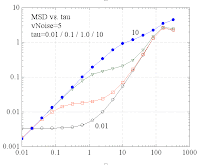## Wednesday, June 18, 2008

### Weak Bead-CSK-Links

Assume there is a preexisting CSK stressfiber network with a particular node (position r_N(t)). The node is performing a random motion with a fractional, superdiffusive MSD. A bead (position r_B(t)) is floating in a viscous medium (friction coefficient gamma), where it experiences white noise thermal forces (amplitude vN of velocity fluctuations), causing a diffusive motion. Now a weak spring (constant k) is connecting the bead to the CSK node. The visco-elastic relaxation time is tau=gamma/k. How does the MSD of the bead linked elastically to the node look like and how does it depend on vN and tau ?

The total force F(t) acting on the bead is given by:

At any moment, the forces from the node, from friction, and from thermal forces are balanced. Setting F(t)=0 yields a dynamic equation for the bead motion, which was solved numerically (program boreas_1).

Parameters:
NMX=64*1024;
dT=0.01;
plExp=1.5;
difus=5.0;

Here the MSD for small thermal noise:And for larger thermal noise:Note that depending on the binding strength (~1/tau), the combination of the diffusive, plateau-like and fractional superdiffusive powerlaws can produce an apparent sub-diffusive regime at intermediate lag-times.

The following figure shows the individual contributions to the total MSD: